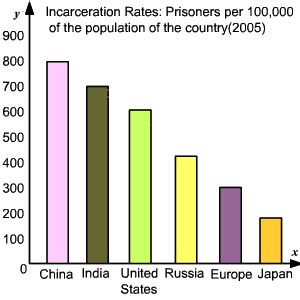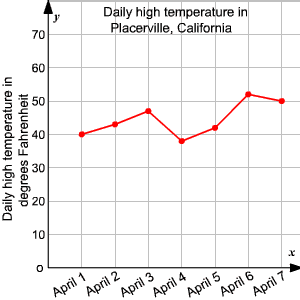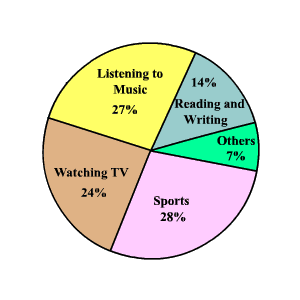# Interpreting Data

Data can be visually represented in many ways. We use bar graphs , line graphs , and circle graphs to present data in a visual way.

### Bar Graphs

A bar graph is used to display the frequency of data in categories , using vertical or horizontal bars. You can easily compare the categories using a bar graph. The bar graph below shows the incarceration rates of different countries. Prisoners per $100,000$ of the population of the country( $2005$ ).### Line Graphs

Line graphs usually show how values change over a period of time . A line graph displays data using line segments.

The line graph below shows the daily high temperature of Placerville , California recorded over a week.### Circle Graphs

Circle graph is used to compare parts of the data to the whole . The entire circle represents $100%$ of the data and each sector represents a percent of the total.

The circle graph below shows the results of a survey that asked teen hobbies, ages $13$ to $19$ .